# Salient Target Detection Method of Video Images Based on Convolution Neural Network

Salient Target Detection Method of Video Images Based on Convolution Neural Network
School of Mathematics and Statistics, Beihua University, Jilin 132013, China

Corresponding Author Email:
guoli@beihua.edu.cn
Page:
2157-2163
|
DOI:
https://doi.org/10.18280/ts.390629
2 August 2022
|
Accepted:
18 October 2022
|
Published:
31 December 2022
| Citation

OPEN ACCESS

Abstract:

Traditional target detection of video images is often used to distinguish the relevant classification of large categories of targets, in the case of complex and diverse image content, it cannot capture enough visual cues, which makes it difficult to distinguish small differences between categories. Therefore, this article studies the salient target detection method of video images based on convolution neural network. Based on dictionary learning, the dynamic features of videos are extracted, and then the coefficient matrix is generated based on the dictionary to complete the learning, so as to realize the complete description of the underlying dynamics of videos. DMD algorithm is used to extract the dynamic mode of videos, and finally the foreground and background of video image frames are separated. Based on YOLOv4 network model, the salient target detection model of video images is constructed. Aiming at the defects of YOLOv4 network model, such as redundant parameters, many convolution modules and complex architecture, a series of model optimization are carried out. Experimental results verify the effectiveness of the model.

Keywords:

convolution neural network, video images, salient target detection

1. Introduction

With the development of computer technology and the continuous improvement of CPU computing power, video image processing technology, which can realize video image enhancement and restoration, target recognition and positioning, is also developing rapidly [1-4]. Video image salient target detection is to simulate human visual perception system, intelligently detect salient targets in video images from semantic level, and finally realize independent analysis and understanding of video image content [5-11]. Traditional target detection of video images is often used to distinguish the relevant classification of large categories of targets, in the case of complex and diverse image content, it can not capture enough visual cues, which makes it difficult to distinguish small differences between categories [12-22]. To solve this problem, it’s impossible to rely on all kinds of artificial image annotation to prompt which areas the detection model needs to extract which target feature information. The correct way to solve the problem is to analyze different subcategories under the same target category and then further analyze the high-level semantic content of video images.

Hu et al.  designed a photoelectric neural network for detecting video objects from long exposure blurred images. The network combines optical encoder, convolutional neural network decoder, and object detection modules, which are optimized end to end. Through back propagation, according to the physical constraints of hardware, the network is updated by joint loss. Simulations and experiments show that the framework can successfully retrieve object labels and bounding boxes at different times of long exposure. Chen and Lang  designed a new interleaving architecture combining 2D convolution network and 3D time network. In order to explore the inter-frame information, a feature aggregation based on time network is proposed. TemporalNet uses appearance-preserving 3D convolution to extract alignment features in the time dimension. The time network runs on multiple scales for better performance. YOLOv4 is used in Naik et al.  for multi-target detection in images and videos, for traffic monitoring applications trained using custom datasets created using Indian road traffic images. The proposed custom model, which has been trained with custom image datasets for 500 periods, achieves 92% training mAP and 0.001 training loss. Fujitake and Sugimoto  proposes a video representation learning framework for real-time video object detection. The proposed framework applies random video prediction to object detection in order to obtain the prior knowledge of videos and then obtain the video representation, and then adjust it to object detection to improve the accuracy. A large number of experiments show that this method uses ResNet-50 on commercial GPU to achieve 73.1% mAP at the speed of 54 frames per second.

In order to obtain a salient target detection model with high precision, high efficiency, low delay and low power consumption, and further expand the application scenarios of target detection algorithm based on deep learning, this article has carried out related research. In Chapter 2, the dynamic features of video are extracted based on dictionary learning, and then the coefficient matrix is generated based on the dictionary to complete the learning, so as to realize the complete description of the underlying dynamics of videos. DMD algorithm is used to extract the dynamic mode of videos, and finally the foreground and background of video image frames are separated. In Chapter 3, the salient target detection model of video images is constructed based on YOLOv4 network model. Aiming at the defects of YOLOv4 network model, such as redundant parameters, many convolution modules and complex architecture, a series of model optimization are carried out. Experimental results verify the effectiveness of the model.

2. Video Foreground Segmentation

If there is no complete background frame in the video to be processed, it will be difficult to detect its salient target. Before target detection, this article extracts the dynamic features of the video based on dictionary learning. That is to say, the dictionary first learns the random patch based on the frame sequence of the input video image, and realizes the infinite approximation of the input video signal. Then, the coefficient matrix is generated based on the dictionary that completes the learning, and the complete description of the underlying dynamics in the video is realized. Figure 1 shows a diagram of dictionary learning and coefficient matrix estimation.

## 1.png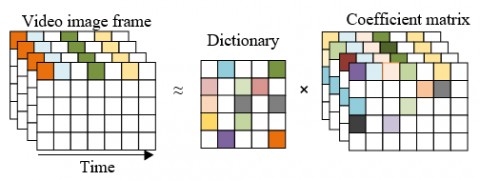Figure 1. Diagram of dictionary learning and coefficient matrix estimation

The goal of dictionary learning random patches based on input video image frame sequence is to extract the most essential features of salient targets in video images. Dictionary learning can reduce the dimension of salient target information in video images, and reduce the interference of redundant information of the target.

The dictionary can be evaluated by the sparsity of the model. The better the dictionary is, the sparser the coefficient matrix is, which requires that the extracted target features are the most important and critical. Assuming that the input video image dimension is cF and the sparse matrix dimension is L, the dictionary model dimension is cF *L; the input video image of sparse matrix is represented by a, the dictionary model is represented by C, and the sparse matrix is represented by β, then:

$a=C \cdot \beta$     (1)

Assuming that the square after the square of each component vector of β is represented by ||β||, the ultimate goal of dictionary learning is to extract the minimum value of ||β||. The problem of extracting dictionary model C can be regarded as an optimization problem to ensure that C and βi can reconstruct ai well and make βi as sparse as possible, that is, to meet the requirements of less distortion and less coupling of dictionary construction.

$\min _{C, \beta_i} \sum_{i=1}^M\left\|a_i-C \cdot \beta_i\right\|_2^2+\mu \sum_{i=1}^M\left\|\beta_i\right\|_1$     (2)

m samples are randomly selected from the video image frame sample set A as the initial value of the dictionary model C, set β=0, and complete the model initialization.

The process of solving ai is illustrated by taking a single sample as an example. Assume that the sample is a vector and the sparse code is β vector. Because a and C are known, solving β under the condition that β is as sparse as possible means minimizing the existence of non-zero elements. First, the element closest to a is extracted. The preliminary β containing the weight of the element is extracted. Based on the weight value of the element, a residual vector, i.e. a preset threshold vector, is extracted. When a' is less than the preset threshold, the calculation is stopped. If it is greater than the preset threshold, go to the next step. Calculate the remaining elements closest to the residual vector a', and then update β to obtain a new residual vector.

All βi can be obtained based on the above steps. Keep β unchanged and update C based on the same step. Then keep C unchanged and update β based on the same steps; obtain the updated dictionary model C by repeating the above process.

Assuming that the number of rows of the sequence matrix and the coefficient matrix of the video image frame are represented by M and L, respectively, for j=1, 2,..., O, the color block representation along all the sequences of the video image frame is represented by W={wij}Ei=1, satisfying the condition of W$\in$RM×O. The coefficient matrices are expressed by Y1={γ*1i.1, γ*1i.2,…, γ*1i.O-1}Ei=1 and Y2={γ*2i.1, γ*2i.2,…, γ*2i.O-1}Ei=1, satisfying Y1, Y2$\in$RM×(O-1). Suppose that the column vector containing overlapping patch E is represented by {.}Ei=1, and the coefficient matrix of the approximate image sequence block is represented by W1={wi.1, wi.2,..., wi.O-1}Ei=1 and W2={wi.2, wi.2,..., wi.O}Ei=1, satisfying W1, W2$\in$RM×(O-1). The regularization parameters used to control the sparsity of the coefficient matrices Y1 and Y2 are represented by μ1 and μ2, respectively. Based on Y1 and Y2, W1 and W2 can be obtained through dictionary learning training.

All the above approximations can be obtained based on solving the minimization problem of the following formula:

$\gamma_{i, j}^{* 1}=\underset{\gamma_{i, j}^1}{\arg \min }\left\|w_{i, j}-C \gamma_{i, j}^1\right\|^2+\mu_1\left\|\gamma_{i, j}^1\right\|_1$

$(i=1,2, \ldots, E, j=1,2, \ldots, O-1)$     (3)

$\gamma_{i, j}^{* 2}=\underset{\gamma_{i,(j-1)}^2}{\arg \min }\left\|w_{i, j}-C \gamma_{i,(j-1)}^2\right\|^2+\mu_2\left\|\gamma_{i,(j-1)}^2\right\|_1$

$(i=1,2, \ldots, E, j=1,2, \ldots, O)$      (4)

## 2.pngFigure 2. Video dynamic mode extraction diagram

Next, DMD algorithm is used to extract video dynamic modes based on Y1 and Y2. Figure 2 shows a video dynamic mode extraction diagram. Set a set of dynamic modes represented by Ψ={ψ1,..., ψs}, the corresponding eigenvalues represented by Δ = {Δ1Δs} and the number of dynamic mode eigenvectors used represented by s, the video image sequence is reconstructed based on Ψ and Δ. In a video image frame, with the change of time point o$\in${0,1,2,...,O-1}, the target will have the associated continuous time-frequency characteristics, and the performance of the target is Ψ. The time-frequency characteristic θj can be calculated by the following formula:

$\theta_j=\frac{\log \left(\Delta_j\right)}{\Delta o}$     (5)

Assuming that the column vector of the j-th dynamic mode containing spatial structure information is represented by ψj and the initial amplitude of the corresponding DMD mode is represented by βj, the approximate video image frame reconstructed at any time point can be obtained by the following formula:

$Y(o) \approx \sum_{j=1}^s \psi_j p^{\theta_j o} \beta_j=\Psi p^{\chi o} \beta$    (6)

The initial vector of β can be obtained based on the initial video image frame, which avoids the trouble in the calculation of {γ*1i.1}Ei=1=Ψβ. Since the eigenvector matrix corresponding to eigenvalues is not a square matrix, then:

$\beta=\Psi^{+}\left\{\tilde{\gamma}_{i, 1}^1\right\}_{i=1}^E$     (7)

In order to separate the foreground and background of a video image frame, the threshold value of low frequency dynamic mode should be processed based on the eigenvalue. In general, the background of an image is stable between successive image frames, and when e$\in${1,2,...,s}, |θe| is about 0. It is assumed that the background of the video image frame is represented by ψepθeoβe, the foreground is represented by ∑j=eψeeoβe, the reconstructed coefficient matrix is represented by Y*={γ*1i.1, γ*1i.2,..., γ*1i.O}Ei=1, and the time index to the O-1 frame is represented by o={0,1,2,...,O-1}. Background separation can be completed by reconstructing video image frames based on the following formula:

$\tilde{Y} \approx \psi_e p^{\theta_e o_{\beta e}}+\sum_{j=e} \psi_j p^{\theta_j \beta_j}$      (8)

The initial amplitude of background is βj=ψ+j{γ*1i.1}Ei=1, and the static background βj at all future time points is constant, which is also the initial amplitude of dynamic foreground. The following formula gives the dictionary reconstruction formula for the fully flattened approximate video image frame sequence B:

$\left\{\tilde{b}_{i, j}\right\}_{i=1, j=1}^{E, O}=C\left\{\tilde{\gamma}_{i, j}\right\}_{i=1, j=1}^{E, O}$    (9)

The diagram of amplitude evolution with time is given in Figure 3.

## 3.png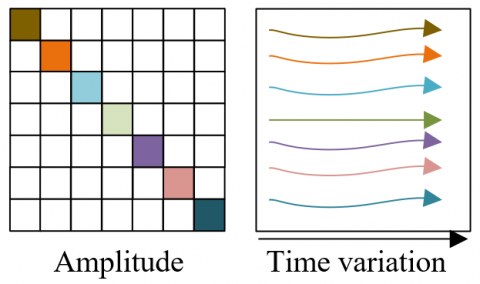Figure 3. Diagram of amplitude evolution with time

3. Optimization of Target Detection Network Model

The salient target detection model of video images constructed in this article is based on YOLOv4 network model. In view of the defects of YOLOv4 network model, such as redundant parameters, many convolution modules and complex architecture, this article optimizes it to improve the efficiency of target detection.

There are many convolution modules in YOLOv4 network model, which affects its forward reasoning efficiency. Therefore, this article firstly optimizes and reconstructs its structure, realizes the lightweight design of the model, and preliminarily optimizes the target detection accuracy. Firstly, it introduces the SPP module, which can effectively enlarge the receptive field of YOLOv4 network and integrate local features and global features.

Set the upward rounding denoted by ⌈⌉, the downward rounding operation denoted by ⌊⌋, the step size denoted by rk, and the feature map size of the video image frame denoted by q. Video image input features will be pooled to the maximum through three cores, which have different sizes and scales. After processing, the features will be spliced by Concat operation, set e=(l-1)/2, and then have the output eigenvalue size calculation formula:

$q^*=\left\lfloor\frac{q+2 e-l}{r_o}\right\rfloor+1$     (10)

The optimized SPP will be set in front of the detection header of the feature network extraction layer.

In order to further simplify the convolution module, this article replaces it with a lightweight Ghost module with high reasoning efficiency and plug and play. Assuming that the number of channels in the input feature map is represented by d and the number of feature mapping is represented by r, it can be seen from the following formula that this method can theoretically improve the reasoning efficiency of r times of the salient target detection model of video images:

$S=\frac{m f^{\prime} q^{\prime} d l l}{m r^{-1} f^{\prime} q^{\prime} d l l+(r-1) m r^{-1} f^{\prime} q^{\prime} c c}=$

$\frac{d l l}{r^{-1} d l l+(r-1) r^{-1} c c}=\frac{r d}{r+d-1} \approx r$        (11)

## 4.png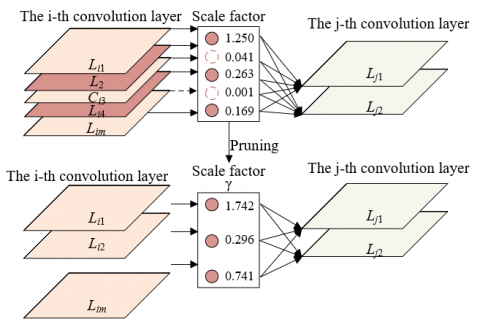Figure 4. Channel pruning diagram

In order to reduce the complexity of the model architecture, this article compresses the parameters of YOLOv4 network model based on the channel pruning limit compression algorithm. Figure 4 shows the channel pruning diagram. Each channel of different convolution layers is matched with a scale factor, and the absolute value of this factor represents the importance of this channel in the operation process. To complete the channel pruning operation, we need to disconnect the connection between the input and output of the target channel first, then carry out sparse training and pruning to make the scale factor gradually approach 0, and finally cut off the channel and connection, which requires the help of

4. Experimental Results and Analysis

# 5.png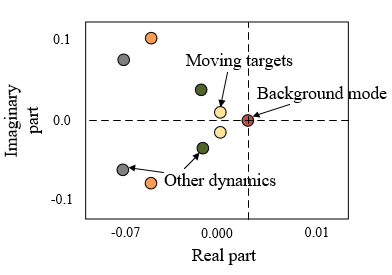Figure 5. The eigenvalues correspond to targets in the video image

Figure 5 shows different eigenvalues of moving targets, background and other dynamic information in video image frames. It can be seen from the figure that the zero eigenvalue near the origin corresponds to the static background, and other dynamics correspond to eigenvalues far from the origin except the moving salient targets.

## 6.png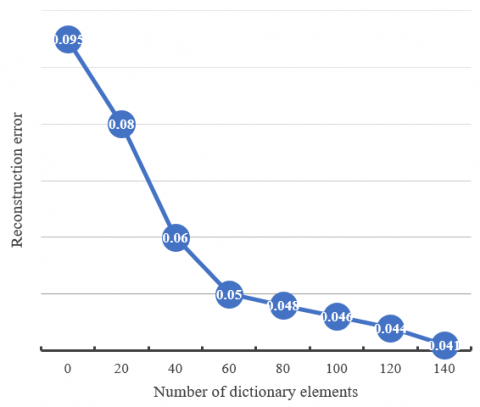Figure 6. Variation of reconstruction error under different number of dictionary elements

The estimation of coefficient matrix determines the approximation of temporal and spatial characteristics of video frames. In order to improve the approximation accuracy, this article solves the equation based on L1 regularization term, and the regularization parameter determines the number of non-zero coefficients which are very important to estimate the signal in sparse matrix. In the video foreground segmentation method, in order to obtain ideal approximate signal, the regularization parameters can be set manually, and the video image sequence can be denoised based on a few dictionary elements. Figure 6 shows the change of reconstruction error under different number of dictionary elements. In this article, we tend to set smaller regularization parameter values to obtain better approximation effect to the input image sequence.

## 7.png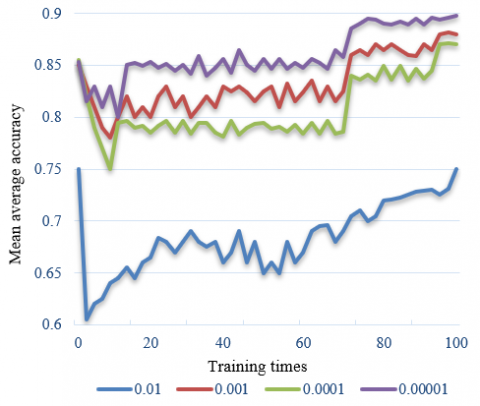Figure 7. Variation of mean average accuracy of different penalty terms

The sparse training of the model needs to set reasonable penalty terms. Figure 7 shows the change of mean average accuracy of different penalty terms. It can be seen from the figure that when the penalty term is 0.0001, the training loss of 100 iterations of the model is the lowest, and the mean average accuracy mAP reaches the highest.

After the sparse training, the model is pruned, and the pruning ratios are set to 0.5, 0.7 and 0.9 respectively. Table 1 shows the influence of different pruning ratios on the model performance.

Table 1. Influence of different pruning rates on model performance

 Model Floating point type operand Model volume Detection accuracy Detection time Device 1 Device 2 Traditional YOLOv4 model 136.25 241.4 94.35 91.24 528.69 Ghost convolution Before module introduction 14.84 162.7 84.26(-2.17) 42.58 217.43 Pruning 0.5 36.29 42.1 81.69(-0.15) 49.62 269.15 Pruning 0.7 11.24 12.5 86.29(-2.64) 35.27 248.37 Pruning 0.9 5.96 5.8 47.69(-41.28) 33.41 185.25

Table 2. Comparison of network performance of target detection model

 Model Floating point type operand Model volume Dataset Detection accuracy Detection time Device 1 Device 2 Before introduction of the SPP module 153.62 23.14 1 76.25 96.25 541.69 2 71.47 - - Before introduction of the Ghost convolution module 64.71 152.64 1 81.2 61.27 347.18 2 82.25 - - Before channel pruning 6.28 25.8 1 89.95 12.35 42.69 2 86.38 - - Final model 3.04 13.6 1 84.42 17.43 33.61 2 82.69 - -

It can be seen from the table that all the performance indexes of the model decrease with the increase of pruning ratio, and the ideal detection effect is achieved on hardware device 1. When the pruning ratio reaches 0.7, the operand of the model is reduced to about 8% of the traditional YOLOv4 model, and the volume is reduced to about 5%. The recognition efficiency on hardware devices 1 and 2 increases to 2.51 times and 2.62 times respectively, and the optimization effect is remarkable. When the pruning ratio continues to rise, the operand and volume of the model are further reduced, but the accuracy of target detection is also lost, and the reasoning efficiency of the model is not ideal. Considering comprehensively, the comprehensive detection performance of the model is the highest when pruning is 0.7.

## 8.png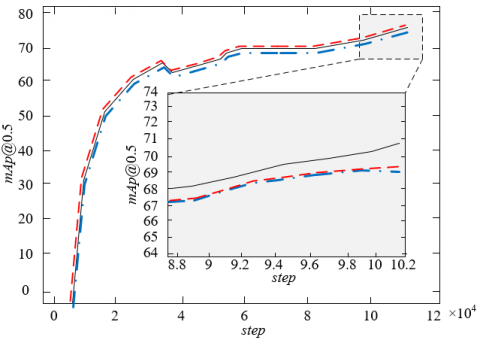Figure 8. Experimental accuracy of salient target detection model in video images

In this article, three cases (i.e., SPP module introduction, Ghost convolution module introduction and the final model) are compared, and model sparse training is carried out respectively. The training times exceed 100,000 times, and the batch size is set to 16. The experimental accuracy of the salient target detection model in video images is shown in Figure 8. As can be seen from the figure, the experimental accuracy of the final model is also obviously improved, increasing by 0.65% with the introduction of SPP module and by 1.34 with the introduction of Ghost convolution module. In addition, the video image reconstructed by the constructed model does not need to be labeled manually, so the detection efficiency is higher. The introduction of Ghost module not only reduces the operand, but also retains abundant feature information of video images. So it is effective to introduce SPP module and Ghost convolution module into the final model constructed in this article.

Table 2 shows the comparison of network performance of target detection models. It can be seen from the table that the final model after module reconstruction and optimization has lower operand and volume, and the time required for target detection of video images is greatly shortened. Floating point type operand is 52.46% of that before the introduction of SPP module and Ghost convolution module, volume is 50.21% of that before the introduction of SPP module and Ghost convolution module, and detection speed is 1.25 times of the original. However, the detection accuracy in dataset 1 decreased slightly by 5.53%, which was mainly caused by channel pruning operation.

5. Conclusion

This article studies the salient target detection method of video images based on convolution neural network. Based on dictionary learning, the dynamic features of videos are extracted, and then the coefficient matrix is generated based on the dictionary to complete the learning, so as to realize the complete description of the underlying dynamics of videos. DMD algorithm is used to extract the dynamic mode of videos, and finally the foreground and background of video image frames are separated. Based on YOLOv4 network model, the salient target detection model of video images is constructed. Aiming at the defects of YOLOv4 network model, such as redundant parameters, many convolution modules and complex architecture, a series of model optimization are carried out. The experimental results show the different features of moving objects, background and other dynamic information in video image frames. The variation of reconstruction error under different number of dictionary elements and the variation of mean average accuracy of different penalty terms are discussed. The influence of different pruning rates on the performance of the model is demonstrated. Three cases (i.e., SPP module introduction, Ghost convolution module introduction and the final model) are compared to verify the effectiveness of the model optimization project.

Acknowledgment

The research is supported by Foundation of Jilin Educational Committee (Grant No.: JJKH20210028KJ); and the Project of Jilin Province Science and Technology Development Plan (Grant No.: YDZJ202201ZYTS648).

References

 Baradaran, M., Bergevin, R. (2022). Object class aware video anomaly detection through image translation. arXiv preprint arXiv:2205.01706. https://doi.org/10.48550/arXiv.2205.01706

 Tian, W.J., Hu, Y.Z. (2021). Label importance ranking with entropy variation complex networks for structured video captioning. Traitement du Signal, 38(4): 937-946. https://doi.org/10.18280/ts.380403

 Mazinani, M.R., Ahmadi, K.D. (2021). An adaptive porn video detection based on consecutive frames using deep learning. Revue d'Intelligence Artificielle, 35(4): 281-290. https://doi.org/10.18280/ria.350402

 Amaria, S., Guidedi, K., Lazarre, W., Kolyang (2022). A Survey on Multimedia Ontologies for a Semantic Annotation of Cinematographic Resources for the Web of Data. Acadlore Transactions on AI and Machine Learning, 1(1): 2-10. https://doi.org/10.56578/ataiml010102

 Li, D., Wang, R., Chen, P., Xie, C., Zhou, Q., Jia, X. (2021). Visual feature learning on video object and human action detection: A systematic review. Micromachines, 13(1): 72. https://doi.org/10.3390/mi13010072

 Guo, Q. (2020). Detection of head raising rate of students in classroom based on head posture recognition. Traitement du Signal, 37(5): 823-830. https://doi.org/10.18280/ts.370515

 Jiao, L., Zhang, R., Liu, F., Yang, S., Hou, B., Li, L., Tang, X. (2021). New generation deep learning for video object detection: A survey. IEEE Transactions on Neural Networks and Learning Systems, 33(8): 3195-3215. https://doi.org/10.1109/TNNLS.2021.3053249

 Pan, T. (2020). Tracking and extracting action trajectory of athlete based on hierarchical features. Ingénierie des Systèmes d’Information, 25(5): 677-682. https://doi.org/10.18280/isi.250515

 Sun, G., Hou, Z. (2021). Model-free-adaptive-control for moving object detection in RGB video sequence. In 2021 IEEE 10th Data Driven Control and Learning Systems Conference (DDCLS), 1442-1447. https://doi.org/10.1109/DDCLS52934.2021.9455653

 Chumachenko, K., Raitoharju, J., Iosifidis, A., Gabbouj, M. (2021). Ensembling object detectors for image and video data analysis. In ICASSP 2021-2021 IEEE International Conference on Acoustics, Speech and Signal Processing (ICASSP), 1515-1519. https://doi.org/10.1109/ICASSP39728.2021.9414013

 Huang, H. (2022). A fast CU depth decision algorithm based on moving object detection for high efficiency video coding. In 2022 2nd International Conference on Consumer Electronics and Computer Engineering (ICCECE), 751-754. https://doi.org/10.1109/ICCECE54139.2022.9712736

 Fischer, K., Fleckenstein, F., Herglotz, C., Kaup, A. (2021). Saliency-driven versatile video coding for neural object detection. In ICASSP 2021-2021 IEEE International Conference on Acoustics, Speech and Signal Processing (ICASSP), 1505-1509. https://doi.org/10.1109/ICASSP39728.2021.9415048

 Guo, S., Zhao, C., Wang, G., Yang, J., Yang, S. (2022). EC2Detect: Real-time online video object detection in edge-cloud collaborative IoT. IEEE Internet of Things Journal, 9(20): 20382-20392. https://doi.org/10.1109/JIOT.2022.3173685

 Muralidhara, S., Hashmi, K.A., Pagani, A., Liwicki, M., Stricker, D., Afzal, M.Z. (2022). Attention-guided disentangled feature aggregation for video object detection. Sensors, 22(21): 8583. https://doi.org/10.3390/s22218583

 Zuo, J., Jia, Z., Yang, J., Kasabov, N. (2020). Moving object detection in video sequence images based on an improved visual background extraction algorithm. Multimedia Tools and Applications, 79(39): 29663-29684. https://doi.org/10.1007/s11042-020-09530-0

 Alpatov, B.A., Babayan, P.V., Ershov, M.D. (2020). Approaches to moving object detection and parameter estimation in a video sequence for the transport analysis system. Computer Optics, 44(5): 746-756. https://doi.org/10.18287/2412-6179-CO-701

 Thenmozhi, T., Kalpana, A.M. (2020). Adaptive motion estimation and sequential outline separation based moving object detection in video surveillance system. Microprocessors and Microsystems, 76: 103084. https://doi.org/10.1016/j.micpro.2020.103084

 Xie, J.C., Xi, R., Chang, D.F. (2022). Mask Wearing Detection Based on YOLOv5 Target Detection Algorithm under COVID-19. Acadlore Transactions on AI and Machine Learning, 1(1): 40-51. https://doi.org/10.56578/ataiml010106

 Xu, D., Xie, W., Zisserman, A. (2019). Geometry-aware video object detection for static cameras. arXiv preprint arXiv:1909.03140. https://doi.org/10.48550/arXiv.1909.03140

 Shokri, M., Harati, A., Taba, K. (2020). Salient object detection in video using deep non-local neural networks. Journal of Visual Communication and Image Representation, 68: 102769. https://doi.org/10.1016/j.jvcir.2020.102769

 Rantelobo, K., Lami, H.F., Louk, A.C., Sastra, N.P. (2020). The object detection on video transmission over wireless visual sensor network. In 2020 3rd International Conference on Mechanical, Electronics, Computer, and Industrial Technology (MECnIT), 54-57. https://doi.org/10.1109/MECnIT48290.2020.9166680

 Lin, G., Fan, W. (2020). Unsupervised video object segmentation based on mixture models and saliency detection. Neural Processing Letters, 51(1): 657-674. https://doi.org/10.1007/s11063-019-10110-z

 Hu, C., Huang, H., Chen, M., Yang, S., Chen, H. (2021). Video object detection from one single image through opto-electronic neural network. APL Photonics, 6(4): 046104. https://doi.org/10.1063/5.0040424

 Chen, M., Lang, J. (2022). TemporalNet: Real-time 2D-3D Video Object Detection. In 2022 19th Conference on Robots and Vision (CRV), 205-212. https://doi.org/10.1109/CRV55824.2022.00034

 Naik, U.P., Rajesh, V., Kumar, R. (2021). Implementation of YOLOv4 algorithm for multiple object detection in image and video dataset using deep learning and artificial intelligence for urban traffic video surveillance application. In 2021 Fourth International Conference on Electrical, Computer and Communication Technologies (ICECCT), 1-6. https://doi.org/10.1109/ICECCT52121.2021.9616625

 Fujitake, M., Sugimoto, A. (2022). Video representation learning through prediction for online object detection. In Proceedings of the IEEE/CVF Winter Conference on Applications of Computer Vision, pp. 530-539.